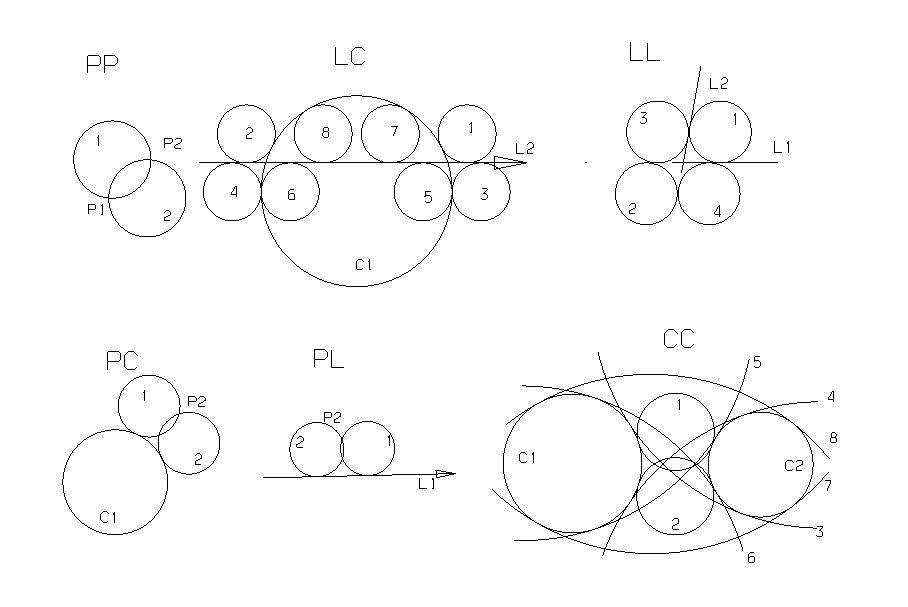```

Also see Circle codes

Circles use shortcut key C (Circle)

Circle from centre, radius [Arc Vector]

# Circle (360-degree)
C20 = P20 VAL(25) DZ

CIR Axis Point
Circle from centerline and point on circle.

Axis-centerline     centerline of new circle.
Point on Circle

# Center, tangential to line or circle
C23=L(P(0 0 0) DX) P(10 10 0)

CIR Cen Tang
Circle from center, tangential to element.
Centerpoint   center of new circle.
Tang          tangent-element:    point, line, circle or polygon;
line or circle in CCV.
[Version]     only for tangent to circle: 1=small circle, 2=big circle.

# Center, tangential to line or circle
C1 = P1 L1
C1 = P1 C1

Circles, tangential to two elements, with a fixed radius and position indication.
Element: (currently only) point, line or arc.
Version: 1 - 8 (there are up to 8 solutions. Select with PgUp/PgDown keys).# Tangential to 2 elements, radius.
# C1 = P1, L1, Radius, Side
# C2 = P1, C1, Radius, Side
# C5 = L1, L2, Radius, Side
# C5 = L1, C1, Radius, Side
# C5 = C1, C2, Radius, Side
L1 = X(10)
L2 = Y(10)
C1 = L1 L2 VAL(5) MOD(1)

# 2 points on the circumference, radius.
# C1 = P1 P2 radius side Z-Axis Vector
P20 = P(98.6 5.4 0)
P21 =  (114.5 -15.2 0)
C22 = P20 P21 VAL(12) MOD(1) DZ

# Point on the perimeter, tangent, radius.
# C1 = P1 L1 radius side
L20 = P (44.6 -32.8 0) P (86.5 -69.3 0)
P26 = P (56.2 -35.5 0)
C21 = P26 L20 VAL (8) MOD (2)

# Point on the perimeter, tangent to a circle.
# C2 = P1 C1 radius side
C20 = P(114.5 -15.2 0) VAL(12) DZ
P27 = P(96.9 -19.7 0)
C21 = P27 C20 VAL(8) MOD(1)

CIR Tang Tang Tang [Version]
Circle tangential to 3 elements.
Element: (currently only) point, line or arc.
Version: 1 - 8 (there are up to 8 solutions; PgUp/PgDown keys).

Entry points must exactly on an input element.

# Circle between 3 lines
C20 = L20 L21 L22

ARC Cen Ang1 Ang2 Radius   [Direction of Rotation]
Circular arc by center, start angle, end angle and radius.
Direction of Rotation: CW shows clockwise, the default is CCW (counter clockwise).

# Central quadrant, R = 10, clockwise.
C21 = ARC P20 ANG(0) ANG(90) 10 CW

ARC Cen PT Angle [Z-Axis] ARC
Arc through centre, starting point and opening angle.
Arc vector is optional.

# Focal starting point quarter
C21 = ARC P20 P21 ANG(90) DZ

ARC PT PT Radius [Z-Axis] [CW/CCW] [Version]      ARC
Circular arc by starting point, end point and radius.
Z-axis-vector is optional.
Version 1 and Default = openingAngle 0-180 degree
Version 2 = openingAngle 180-360 degree

# Arc from start point to endpoint radius arc vector
C21 = ARC P(251 23 0) P(189 75 0) VAL(50) DZ

ARC PT Tang Radius [Angle] [Z-Axis] [CW/CCW]    ARC
Circular arc by starting point, starting direction and radius.
Starting direction: the tangent-direction to the circle in the starting point.
Vector, line, circle, polygon, ellipsis, b-spline, clothoid.
Angle: opening-angle, optional. Default is 360 degrees.
Axis-vector: optional. Invert vector to change side (eg "DIZ").
[CW/CCW] is the direction of rotation. Change with PageUp-key.

# Example: Final tangent of a clothoid (spiral-form curve) as a starting direction.
S20 = CLOT P(0 0 0) DX ANG(30) 0 10
P20 = P(S20 1)
D20 = TNG P20 S20
C20 = ARC P20 D20 25 ANG(30)

ARC PT PT Cen [Z-Axis] [CW/CCW]      ARC
Circular arc by starting point, end point and center
Arc vector is optional.
[CW/CCW] is the direction of rotation. Default is "CW".
C20=ARC P(10,6) P(16 0) DIZ

ARC PT PT PT  [CW/CCW]         ARC1
Arc by three points on the perimeter.
[CW/CCW] is the direction of rotation. Default is "CW".

C20 = ARC1 P(0 0) P(10 0) P(10 10)

-------------------------------------------------- ----------------
Others:

# change sense of rotaion of existing circle
C2=C1,REV

```